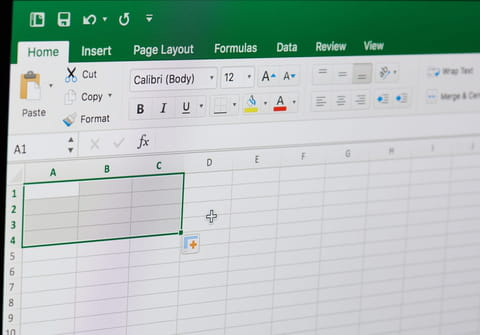# Create a new workbook and copy data in Excel via a MacroOften we need to carry out manipulations in Excel documents and apply them to multiple rows, columns and sheets. To do this manually takes too much time and allows for errors to creep in, so Excel offers the option of Macros, which carry out data manipulation operations for you. In this article we will show you how to use a pre-existing Macro to create a new workbook and copy data into a new spreadsheet.

## How to use a macro to create a new workbook and copy data?

1. Make a backup of the spreadsheet.

2. Open the workbook.

3. Press ALT + F11 to open VBE.

4. Go to Insert > Module.

5. Copy and paste the following code into the module:

```Sub details()
Dim thisWB  As String
Dim newWB As String
thisWB = ActiveWorkbook.Name
On Error Resume Next
Sheets("tempsheet").Delete
On Error GoTo 0
ActiveSheet.Name = "tempsheet"
Sheets("Sheet1").Select
If ActiveSheet.AutoFilterMode Then
Cells.Select
On Error Resume Next
ActiveSheet.ShowAllData
On Error GoTo 0
End If
Columns("B:B").Select
Selection.Copy
Sheets("tempsheet").Select
Range("A1").Select
ActiveSheet.Paste
Application.CutCopyMode = False
If (Cells(1, 1) = "") Then
lastrow = Cells(1, 1).End(xlDown).Row
If lastrow <> Rows.Count Then
Range("A1:A" & lastrow - 1).Select
Selection.Delete Shift:=xlUp
End If
End If
Columns("A:A").Select
CopyToRange:=Range("B1"), Unique:=True
Columns("A:A").Delete
Cells.Select
Selection.Sort _
Key1:=Range("A2"), Order1:=xlAscending, _
MatchCase:=False, Orientation:=xlTopToBottom, _
DataOption1:=xlSortNormal
lMaxSupp = Cells(Rows.Count, 1).End(xlUp).Row
For suppno = 2 To lMaxSupp
Windows(thisWB).Activate
supName = Sheets("tempsheet").Range("A" & suppno)
If supName <> "" Then
ActiveWorkbook.SaveAs supName
newWB = ActiveWorkbook.Name
Windows(thisWB).Activate
Sheets("Sheet1").Select
Cells.Select
If ActiveSheet.AutoFilterMode = False Then
Selection.AutoFilter
End If
Selection.AutoFilter Field:=2, Criteria1:="=" & supName, _
Operator:=xlAnd, Criteria2:="<>"
lastrow = Cells(Rows.Count, 2).End(xlUp).Row
Rows("1:" & lastrow).Copy
Windows(newWB).Activate
ActiveSheet.Paste
ActiveWorkbook.Save
ActiveWorkbook.Close
End If
Next
Sheets("tempsheet").Delete
Sheets("Sheet1").Select
If ActiveSheet.AutoFilterMode Then
Cells.Select
ActiveSheet.ShowAllData
End If
End Sub```

6. Press F5 to run the macro.

7. Check if it has worked.

Do you need more help with excel? Check out our forum!
Subject Replies